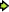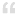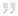## Saturday, December 20, 2008

### Spin Images at Web sites Use of Simple Java codeThere are lots of Java secrets out there but still many of secret have to discuses. In this site I have already discussed some of them but today I thought to be slightly different and giving you a special java code that is effect on images of any web page.

Normally this is injecting on any site images but if you need eye catching demonstration by this code then should consider for sites that has more and small size images. Thumbnail view of images makes more spin than larger set of images.

For the purpose of testing you can use below sites and check the behavior of this :http://www.images.google.comhttp://www.flickr.com/explore/interesting/2008/10/

Through out this I'm using Google image search but this is for explanation purpose only.javascript:R= 0; x1=.1; y1=.05; x2=.25; y2=.24; x3=1.6; y3=.24; x4=300; y4=200; x5=300; y5=200; DI= document.images ; DIL=DI.length; function A(){for(i=0; i<DIL; i++){DIS=DI[ i ].style; DIS.position='absolute'; DIS.left=Math. sin(R*x1+ i*x2+x3)* x4+x5; DIS.top=Math. cos(R*y1+ i*y2+y3)* y4+y5}R++ }setInterval('A()',5); void(0)